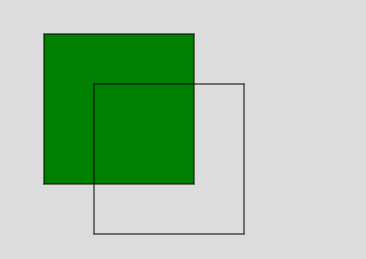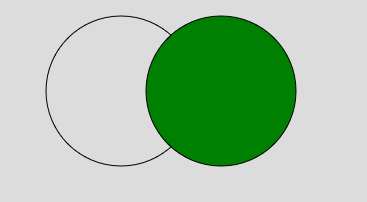Open In App
Related Articles
• Write an Interview Experience
• p5.js
• p5.js Introduction

# p5.js fill() Function

p5.js fill() function is used to fill the color of the shapes. This function supports all types of color objects. For example RGB, RGBA, Hex CSS color, and all named color strings. The color object can also be set as a string in terms of RGB, RGBA, Hex CSS color, or a named color string.

Syntax:

```fill( v1, v2, v3, alpha )
or
fill( value )
or
fill( gray, alpha )
or
fill( values )
or
fill( color )```

Parameters:

• v1: It is used to set the red or hue value relative to the current color range.
• v2: It is used to set the green or saturation value relative to current color range.
• v3: It is used to set the blue or brightness value relative to current color range.
• alpha: It is used to set the transparency of the drawing.
• value: It is used to set the value of a color string.
• gray: It is used to set the gray value.
• values: It is an array containing the red, green, blue, and alpha values.
• color: It is used to set the stroke color.

Below examples illustrate the fill() function in p5.js:

Example 1:

## javascript

 `function` `setup() { ``     ` `    ``// Create Canvas of given size ``    ``createCanvas(400, 300); ``} `` ` `function` `draw() { ``     ` `    ``// Set the background color ``    ``background(220); ``     ` `    ``// Use fill() function to fill color``    ``fill(``'green'``)``    ``// Draw a line ``    ``rect(50, 50, 150, 150); ``     ` `    ``// Use noFill() function``    ``noFill();``   ` `    ``// Draw a line ``    ``rect(100, 100, 150, 150); ``} `

Output:Example 2:

## javascript

 `function` `setup() { ``     ` `    ``// Create Canvas of given size ``    ``createCanvas(400, 300); ``} `` ` `function` `draw() { ``     ` `    ``// Set the background color ``    ``background(220); ``     ` `    ``// Use noFill() function``    ``noFill();``     ` `    ``// Draw a circle ``    ``circle(140, 100, 150);``     ` `    ``// Use fill() function to fill color``    ``fill(``'green'``);``     ` `    ``// Draw a circle ``    ``circle(240, 100, 150); ``} `

Output:Reference: https://p5js.org/reference/#/p5/fill